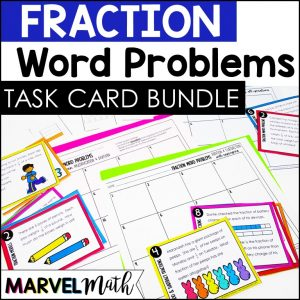Save 10% on your first order with code EXTRA10

# Adding and Subtracting Fractions Word Problems

\$3.00\$5.00
SKU: 7965070 Categories: ,

## Description

Your students will love these Bright and Engaging Fraction Word Problems Task Cards to practice Adding and Subtracting Fractions! In these word problems students will practice adding and subtracting fractions with like and unlike denominators, regrouping fractions while adding and subtracting, and finding information from tables to solve word problems

Save money on this resource by purchasing it in the 5th Grade Fraction Word Problem Bundle!

Topics Covered

• Word Problems
• Adding and Subtracting Fractions with Unlike Denominators
• Adding and Subtracting Fractions with Like Denominators that require Regrouping
• Problems involving Models

What’s included?

• Task Card sized Cover and Key for easy organization
• Student Recording Sheets
• Color and Black and White Versions of Task Cards

How Can I use these?

• Early Finishers
• Tutoring
• Math Stations
• Fraction Review

Common Core Standards Alignment:

5.NF.A.2 Solve word problems involving addition and subtraction of fractions referring to the same whole, including cases of unlike denominators, e.g., by using visual fraction models or equations to represent the problem.

TEKS Alignment:

5.3K Add and subtract positive rational numbers fluently